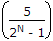# Electronics and Communication Engineering - Digital Electronics

1.
The resolution of an n bit DAC with a maximum input of 5 V is 5 mV. The value of n is
8
9
10
11
Explanation:1000 = 5 or N = 10.

2.
2's complement of binary number 0101 is
1011
1111
1101
1110
Explanation:

1's complement of 0101 is 1010 and 2's complement is 1010+1 = 1011.

3.
An OR gate has 4 inputs. One input is high and the other three are low. The output
is low
is high
is alternately high and low
may be high or low depending on relative magnitude of inputs
Explanation:

In OR any input high means high output.

4.
Decimal number 10 is equal to binary number
1110
1010
1001
1000